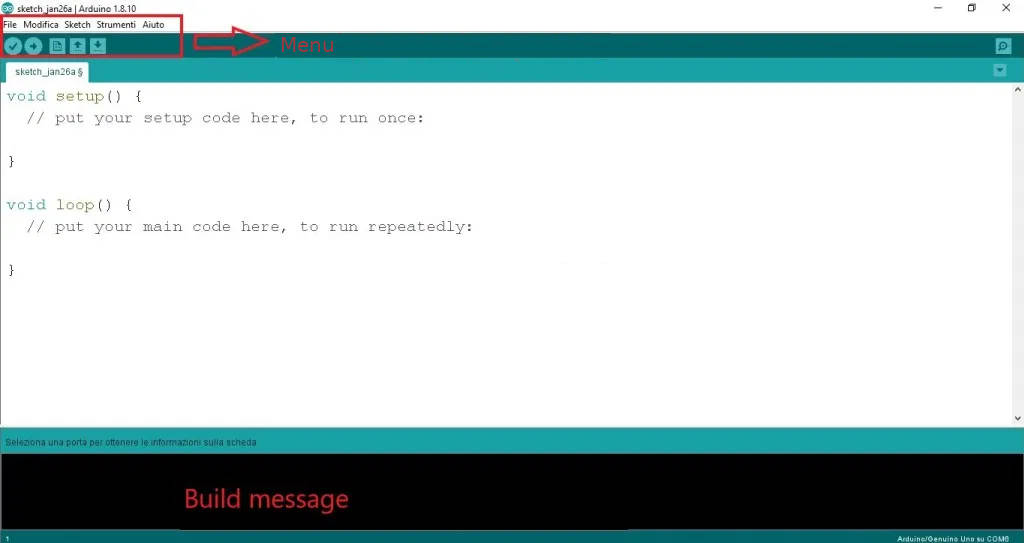# Arduino IDE: functions and RGB LEDs at work #9.1Welcome back to our programming tutorial using the Arduino IDE. Today we will improve our knowledge of functions with practical examples and a RGB LED.

You can take a look at the previous chapters of the course here:

In the previous article of the guide we exposed the theoretical concepts on the behavior of a function.

In this article you will be able to understand how a function works from within the Arduino IDE software.

In the following project, the RGB LED will light up first in red, then in green and finally in blue and then cyclically return back to red.

The following materials are needed to carry out this project:

The connection diagram is as follows:The wiring diagram is as follows:Code with a void function (a detailed explanation is given in the following lines):

The void function is a very useful type of function, since it allows you to carry out various instructions (procedures) based on the number of calls the function made. The behavior of this function is quite self-explanatory when looking at the program.

The second sketch uses functions with a float return type. The purpose of the program is to calculate the value of the voltage applied at a specific terminal on the breadboard. Once calculated, this value will be converted to an 8-bit value (0-255 instead of 0-1023).

The sketch is as follows:

As for the second sketch we can observe the similarity between the declaration, call and definition of the function. The same number of parameters was used.

What happens when a function is called?

As reported in the previous article, the parameter(s) used when calling a function are called current parameters, while the parameters used when defining a function are called formal parameters.

When a function is called in the void loop (or void setup) the value of the current parameter of the function called in question will be assigned to the formal parameter of the defined function. In a nutshell, as regards this sketch, the value of the variable of the current parameter “l” will be assigned to the formal parameter “a” (of the defined function).

How does the return statement behave?

The return statement has a certain argument. This argument is represented by a number or variable. As far as the second sketch is concerned, the return statement has the variable “p” as its argument.

The value of the variable will be assigned to the variable that called the function (in this the value of the variable “p” will be assigned to the variable “l”, which called the function).

Follow us to keep yourself updated with the news!

### Simone CandidoSimone Candido è un ragazzo appassionato del mondo tech nella sua totalità. Simone ama immedesimarsi in nuove esperienze, la sua filosofia si basa sulla irrefrenabile voglia di ampliare a 360° le sue conoscenze abbracciando tutti i campi del sapere, in quanto ritiene che il sapere umano sia il connubio perfetto tra cultura umanistica e scientifica.

This site uses Akismet to reduce spam. Learn how your comment data is processed.